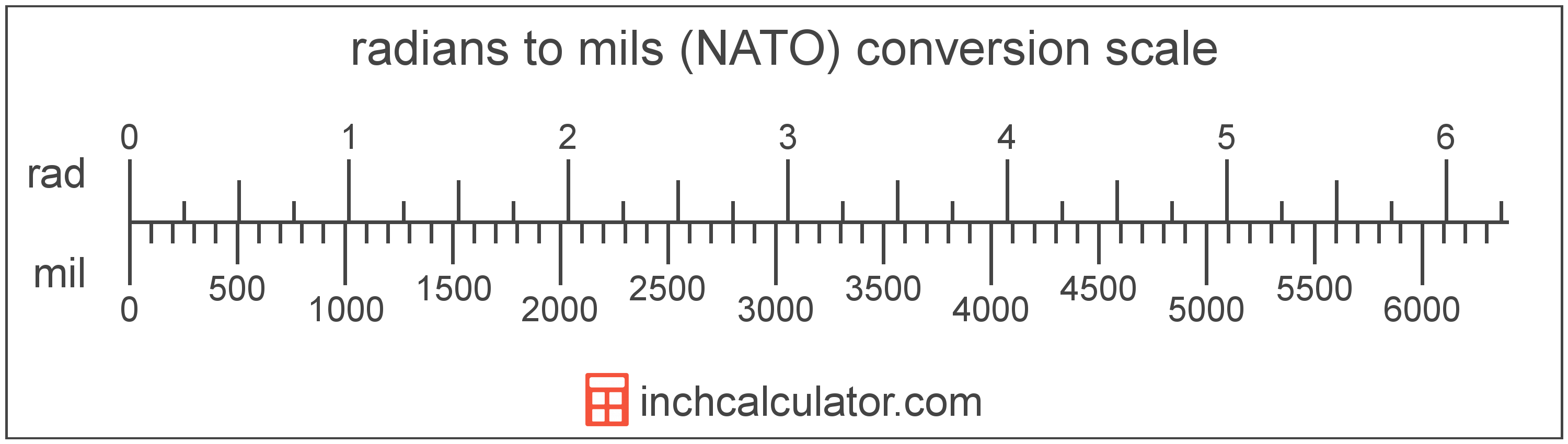# Mils (NATO) to Radians Converter

Enter the angle in mils below to get the value converted to radians.Do you want to convert radians to mils?

## How to Convert Mils to Radians

To convert a measurement in mils to a measurement in radians, multiply the angle by the following conversion ratio: 0.000982 radians/mil.

Since one mil is equal to 0.000982 radians, you can use this simple formula to convert:

The angle in radians is equal to the angle in mils multiplied by 0.000982.

For example, here's how to convert 5,000 mils to radians using the formula above.## What is a Mil?

A mil, short for milliradian, is equal to 1/6,400 of a circle. The mil used by the US military and NATO forces is slightly different than the true value of a milliradian, which is equal to 1/6,283 of a circle.

During World War I the US adopted what is now the NATO mil to replace degrees and minutes for use in artillery sights. They opted to round mils to 6,400 per circle for simplicity at the time. Today, the mil is commonly used to measure adjustment of sights and scopes of firearms.

There is rightfully much confusion as a result of the mil adopted by the US military and NATO being slightly different than the milliradian.

A mil is sometimes also referred to as an angular mil. Mils can be abbreviated as mil; for example, 1 mil can be written as 1 mil.

A radian is the measurement of angle equal to the length of an arc divided by the radius of the circle or arc. 1 radian is equal to 180/π degrees, or about 57.29578°. There are about 6.28318 radians in a circle.

The radian is the SI derived unit for angle in the metric system. Radians can be abbreviated as rad, and are also sometimes abbreviated as c, r, or R. For example, 1 radian can be written as 1 rad, 1 c, 1 r, or 1 R.

Radians are often expressed using their definition. The formula to find an angle in radians is θ = s/r, where the angle in radians θ is equal to the arc length s divided by the radius r. Thus, radians may also be expressed as the formula of arc length over the radius.

Radians are also considered to be a "unitless" unit. That is, when multiplying or dividing by radians, the result does not include radians as part of the final units.

For example, when determining the length of an arc for a given angle, we use the formula above, rearranged to be s = θr. If θ is in radians and r is in meters, then the units of s will be meters, not radian-meters. If θ were in degrees, however, then s would have units of degree-meters.

## Mil to Radian Conversion Table

Table showing various mil measurements converted to radians.